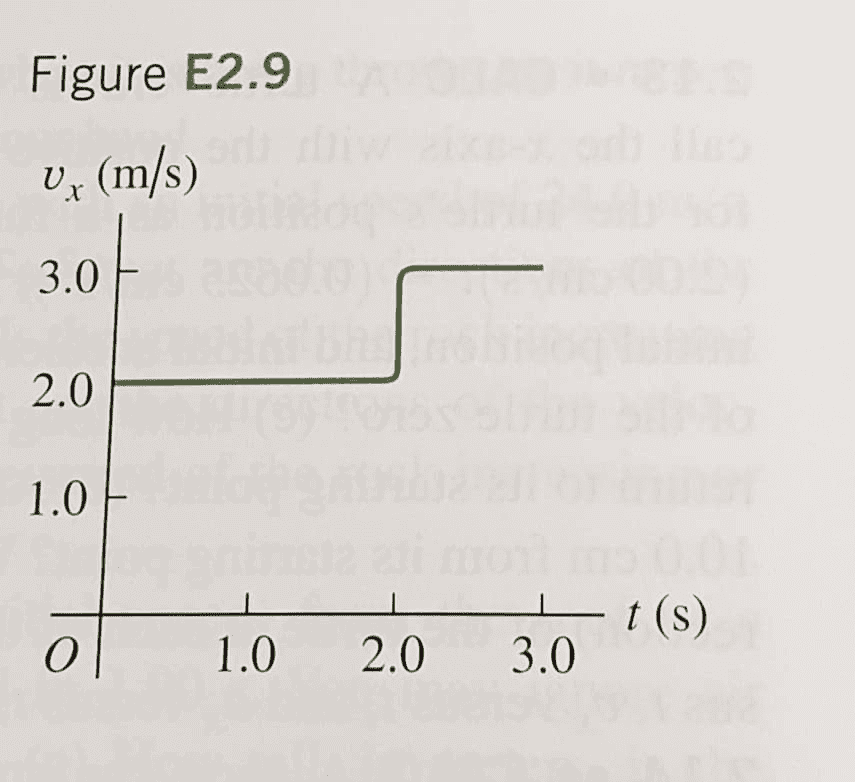# Average speed and average velocity

Homework Statement:
A ball moves in a straight line ( the x-axis). The graph shows this ball's velocity as a function of time.
a) What are the ball's average speed and average velocity during the first 3.0 s?
b) Suppose that the ball moved in such a way that the graph segment after 2.0 s was -3.0 m/s instead of +3.0 m/s. Find the ball's average speed and average velocity in this case.
Relevant Equations:
##v_{av-x}=\frac{v_3+v_0}{2}##So thing that confusses me, is what is the difference regarding the calculation of speed and velocity? In think I should use the formula I wrote above to find the average velocity, but I don't understand what the formula for average speed will be then. By the way, when using the formula above, should I use the values instead of two?

##\frac{v_3+v_2+v_1}{3}=\frac{2+2+3}{3}=2.33 m/s ##

I really appreciate some help! :)

PeroK
Homework Helper
Gold Member
2020 Award
Homework Statement:: A ball moves in a straight line ( the x-axis). The graph shows this ball's velocity as a function of time.
a) What are the ball's average speed and average velocity during the first 3.0 s?
b) Suppose that the ball moved in such a way that the graph segment after 2.0 s was -3.0 m/s instead of +3.0 m/s. Find the ball's average speed and average velocity in this case.
Relevant Equations:: ##v_{av-x}=\frac{v_3+v_0}{2}##

View attachment 259773
So thing that confusses me, is what is the difference regarding the calculation of speed and velocity? In think I should use the formula I wrote above to find the average velocity, but I don't understand what the formula for average speed will be then. By the way, when using the formula above, should I use the values instead of two?

##\frac{v_3+v_2+v_1}{3}=\frac{2+2+3}{3}=2.33 m/s ##

I really appreciate some help! :)
By definition, average speed is total distance travelled over total time; and average velocity is total displacement over total time.

•WWGD and Kolika28
jbriggs444
Homework Helper
"average speed" and "average velocity" are both examples of weighted averages. The only difference is the thing that is being averaged. Speed is always positive (or zero). It adds like an ordinary unsigned number. Velocity has a direction. It adds like a vector. In the one dimensional case, it adds like an ordinary signed number.

For a weighted average, you obviously need a weight. For average speed and average velocity in the physics classroom, the weight is always going to be time unless explicitly specified otherwise. Weighting based on distance can also be useful in some cases, but let us not go there.

The underlying formula for computing a weighted average is$$\frac{v_1w_1 + v_2w_2 + ...}{w_1 + w_2 + ...}$$where the ##v_x## are the values you are averaging and the ##w_x## are the weights.

You take the sum of the weighted values and divide by the sum of the weights. [For an un-weighted average, the weights are all equal to one and you can just sum up the values and divide by the number of values like you learned in grade school].

Note that I use a different definition for "average speed" and "average velocity" than @PeroK. But we agree that the computed average speed and average velocity are the same for either definition. [The fact that they are equal is why time-weighted averages are useful]

Last edited:
•Lnewqban and Kolika28
Thank you guys! I think I understand it now!